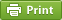Werner's Blog — Opinion, Analysis, Commentary
Fun with indicator variables

In an empirical research paper I came across the authors report mean and standard deviation of a binary (0/1-indicator) variable, or something we commonly refer to as a "dummy variable" in econometrics. I thought that the standard deviation was redundant information, and on closer inspection indeed it is. The mean $$p$$ is of course just the proportion of positive responses ("1"). The standard deviation is a simple transformation of that proportion.

The standard deviation of a sample of $$n$$ observations of the indicator variable $$x_i$$ is very easy to calculate. The mean is defined as $$p=\sum_i x_i/n$$, and then the variance is given by $\sigma^2=\frac{1}{n}\sum_i (x_i-p)^2= \frac{pn(1-p)^2+(1-p)n(0-p)^2}{n}=p(1-p)$ Therefore the sample standard deviation is $\sigma=\sqrt{p(1-p)}$ and the unbiased estimator of the standard deviation is $s=\sqrt{\left(\frac{n}{n-1}\right)p(1-p)}$ A dummy variable with an equal proportion of zero and one responses must therefore have a sample standard deviation of exactly 0.5, and that is the highest it gets. As $$p$$ approaches zero or one, the standard deviation gets smaller and smaller.

So when your software reports standard deviations of dummy variables, please do no repeat it in your "summary statistics" tables. It is completely redundant information.

If you'd like to have a bit more fun with binary variables, here is a simple challenge. What is the sample correlation coefficient of two indicator variables with proportions $$p_x$$ and $$p_y$$ of individual positive responses and proportion $$p_{xy}$$ of joint positive responses? The answer is: $r= \frac{p_{xy}-p_x p_y}{\sqrt{ p_x (1-p_x) p_y (1-p_y) }}$

There are actually some interesting insights in this if you consider the bounding cases. The smallest possible correlation happens when no positive indicator variables match and $$p_{xy}=0$$, which in turn is only possible when $$p_x+p_y\lt 1$$. If the sum of the two proportions exceeds one, then there must be at least $$p_x+p_y-1$$ joint positive responses. It is then easy to show that $r_{\min}=-\sqrt{\min\left\{\frac{p_x}{1-p_x}\frac{p_y}{1-p_y}, \frac{1-p_x}{p_x}\frac{1-p_y}{p_y}\right\}}$ Put another way, if your proportions $$p_x$$ and $$p_y$$ are small, then your correlation cannot be large in magnitude. To obtain a perfect negative correlation of $$-1$$, both proportions must be exactly equal to one half. Similarly, one can determine the largest possible correlation. The maximum number of positive matches is $$\min\{p_x,p_y\}$$. Therefore, it follows that $r_{\max}=+\sqrt{\min\left\{\frac{p_x}{1-p_x}\frac{1-p_y}{p_y}, \frac{1-p_x}{p_x}\frac{p_y}{1-p_y}\right\}}$ A perfect correlation of $$+1$$ is feasible when both proportions are exactly equal, regardless of how large they are.

If you work with dummy variables and you deal with low frequency events where $$p_x$$ and $$p_y$$ are small, getting large positive correlations is perfectly possible. However, getting large negative correlations is nearly impossible. To get large negative correlations, your proportions must be both close to one-half.

Posted on Friday, June 26, 2015 at 07:45 — #EconometricsRecent Blog Entries

Topics

Months

Subscribe to RSS feed© 2023  Prof. Werner Antweiler, University of British Columbia.